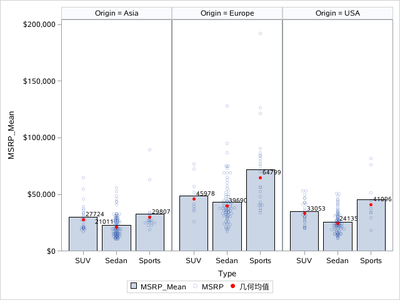## proc sgpanel: is datalabel supposed to work with limitlower and limitupper?

Hello,

I am trying to create a bargraph in which a scatter plot is overlaid with a bar graph. The bar graph is supposed to represent the geometric mean, and the error bars the lower and upper confidence limits of the mean. I used scatter and vbarparm statemens to achieve that, together with a dataset with precomputed values stacked under the raw dataset.

Unfortunately, the vbarparm does not seem to like having datalabel together with limitlower and limitupper, I am getting the following error (not sure where German is coming from, other messages are in English):

Spoiler
NOTE: Die Option DATALABEL= wird im Fehlerbalken nicht unterstützt. Das Etikett wird nicht erstellt.

This is odd as the documentation indirectly implies that the options are compatible:

If you also specify LIMITLOWER or LIMITUPPER, then the limit labels are also shown. Prior to SAS 9.4M5, limit labels are shown when you use DATALABEL or DATALABEL=variable with the limit options. Starting with SAS 9.4M5, limit labels are shown only when you use DATALABEL (without a variable).

Here is the example code:

``````proc summary data=sashelp.cars noprint nway;
var msrp;
class Origin Make;
output out=cars_summary mean= uclm= lclm= /autoname;
run;

data for_plot;
set sashelp.cars cars_summary;
run;

proc sgpanel data=for_plot;
panelby Origin /layout=columnlattice;
vbarparm category=Make response=MSRP_Mean / datalabel limitlower=MSRP_LCLM limitupper=MSRP_UCLM;
scatter x=Make y=MSRP/ jitter;
run;``````

Is this supposed to work? If not, how can I overlay the geometric mean values on top of the panel?

1 ACCEPTED SOLUTION

Accepted Solutions

## Re: proc sgpanel: is datalabel supposed to work with limitlower and limitupper?

It is even simpler, thank you for pointing me towards highlow:

``````proc summary data=sashelp.cars noprint nway;
var msrp;
class Origin Type;
output out=cars_summary mean= uclm= lclm= /autoname;
run;

data for_plot;
set sashelp.cars cars_summary;
run;

proc sgpanel data=for_plot;
panelby Origin /layout=columnlattice;
vbarparm category=Type response=MSRP_Mean / datalabel;
scatter x=Type y=MSRP/ jitter;
highlow x=Type low=MSRP_LCLM high=MSRP_UCLM;
run;``````
4 REPLIES 4Ksharp
Super User

## Re: proc sgpanel: is datalabel supposed to work with limitlower and limitupper?

You want this ?

``````proc summary data=sashelp.cars noprint nway;
var msrp;
class Origin type;
output out=cars_summary mean= uclm= lclm= /autoname;
run;

data for_plot;
set sashelp.cars cars_summary;
run;

proc sgpanel data=for_plot;
panelby Origin /layout=columnlattice;
vbarparm category=type response=MSRP_Mean /name='a';
scatter x=type y=MSRP/ jitter name='b';
highlow x=type low=MSRP_LCLM high=MSRP_UCLM /LOWLABEL=MSRP_LCLM HIGHLABEL=MSRP_UCLM
HIGHCAP=SERIF LOWCAP=SERIF LINEATTRS=(color=red) LABELATTRS=(color=red);
keylegend  'a' 'b';
run;``````## Re: proc sgpanel: is datalabel supposed to work with limitlower and limitupper?

I want the label to show the (geometric) mean, not the confidence limits.Ksharp
Super User

## Re: proc sgpanel: is datalabel supposed to work with limitlower and limitupper?

Why not calculate it and plot it ?

``````proc summary data=sashelp.cars noprint nway;
var msrp;
class Origin type;
output out=cars_summary mean= uclm= lclm= /autoname;
run;
proc univariate data=sashelp.cars noprint outtable=cars_summary2(keep=Origin type _GEOMEAN_);
var msrp;
class Origin type;
run;

data for_plot;
set sashelp.cars cars_summary cars_summary2;
if type=:'S';
run;

proc sgpanel data=for_plot;
panelby Origin /layout=columnlattice;
vbarparm category=type response=MSRP_Mean ;
scatter x=type y=MSRP/ jitter transparency=0.8;
scatter x=type y=_GEOMEAN_/markerattrs=(symbol=circlefilled color=red) datalabel=_GEOMEAN_;
run;``````## Re: proc sgpanel: is datalabel supposed to work with limitlower and limitupper?

It is even simpler, thank you for pointing me towards highlow:

``````proc summary data=sashelp.cars noprint nway;
var msrp;
class Origin Type;
output out=cars_summary mean= uclm= lclm= /autoname;
run;

data for_plot;
set sashelp.cars cars_summary;
run;

proc sgpanel data=for_plot;
panelby Origin /layout=columnlattice;
vbarparm category=Type response=MSRP_Mean / datalabel;
scatter x=Type y=MSRP/ jitter;
highlow x=Type low=MSRP_LCLM high=MSRP_UCLM;
run;``````
Discussion stats
• 4 replies
• 242 views
• 0 likes
• 2 in conversation